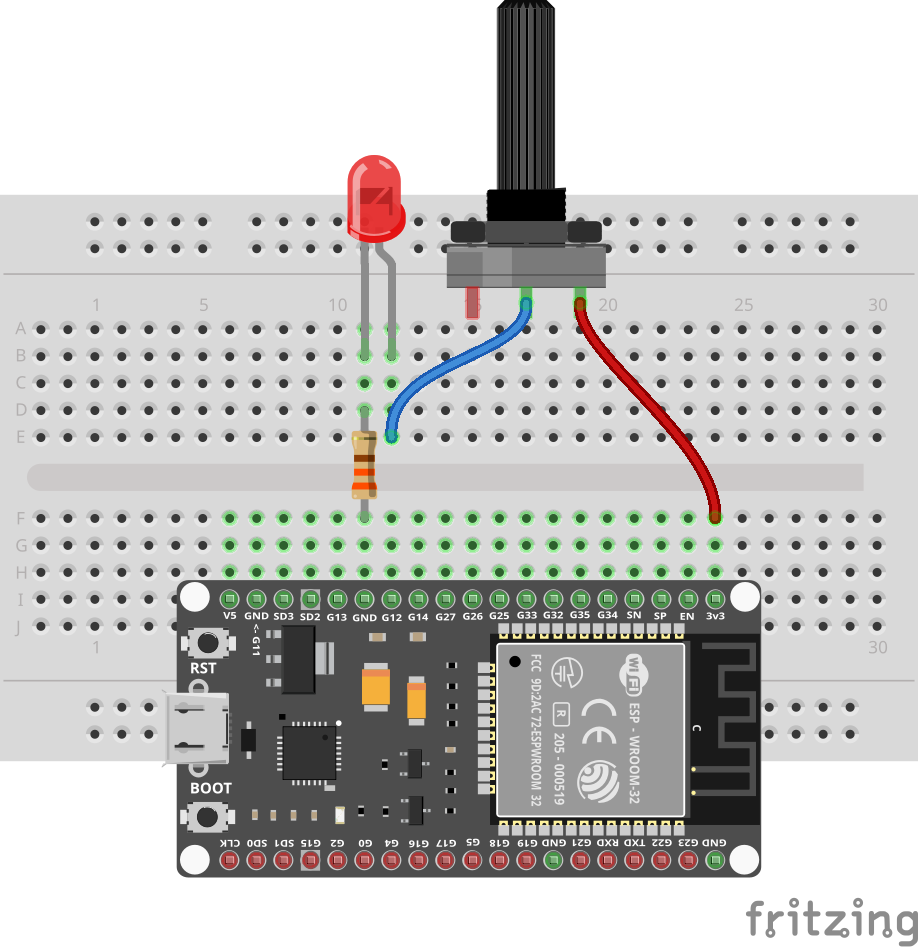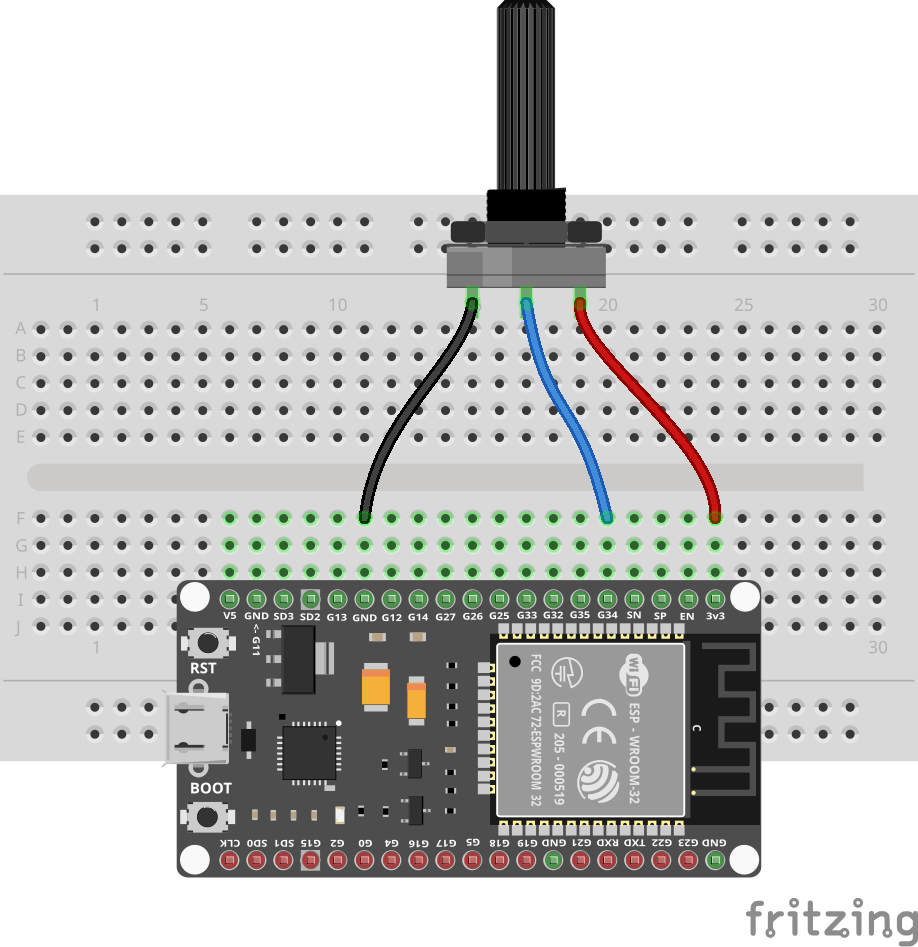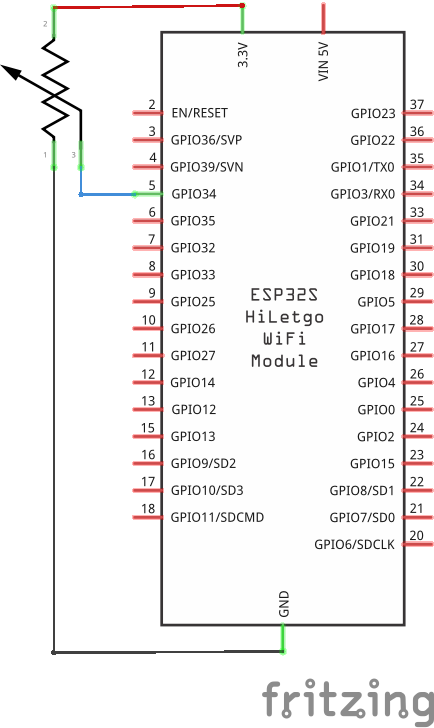# Potentiometer

A potentiometer is a variable resistor with 3 terminals. A so-called "wiper" slides over a resistive element dividing the full resistance based on its position.

The most common potentiometer value is 10kΩ. This means that the resistance between the outer terminals is 10kΩ. The resistance between the wiper and one of the outer terminals is 0Ω when the wiper is at that terminal, and 10kΩ when the wiper is at the other terminal.

## Prerequisites

You should have done the ADC tutorial before this one.

## Variable resistor

Before using the potentiometer together with the ESP32, let's see how it works. Connect the potentiometer as follows:

• VCC (one of the terminals) to 3V3
• OUT (the wiper; typically in the middle) to an LED
• the LED to a 330Ω resistor
• the resistor to GNDIn this case we use the ESP32 board simply as a power supply. The potentiometer is a variable resistor, so we can use it to dim the LED. Turn the knob and see the LED get brighter and dimmer.

In the binary sensors tutorial we mentioned that many binary sensors come with a blue potentiometer to set the sensitivity. This is how it works: the potentiometer is used as a variable resistor to set the threshold for the sensor.

## Setup with a microcontroller

Connect the potentiometer as follows:

• GND (one of the terminals) to GND
• VCC (the other terminal) to 3V3
• OUT (the wiper) to pin 34## Code

You can use the program from the ADC tutorial to see the voltage change when the knob is turned.

```import gpio

main:
pin := gpio.Pin 34